# [PYTHON] [Machine learning] I tried to do something like passing an image

Hello. This is Yuki Ando (@ holiday1173).

In this article, using a type of clustering method called the K-means method introduced in the previous article, [pathization (vectorization)](https: // school) of images, which is often used in Illustrator, etc. .dhw.co.jp/word/design/vector.html) I tried something like that.

## Overview

Here's what I did in the last article:

1. Clustering the pixel value of each pixel of the image using K-means

2. Assign the average pixel value in the cluster for each cluster, reduce the number of colors and draw

Previous article → [Machine learning] Explaining color quantization

Here is the result of the actual processing. (Left: Before processing, Right: After processing)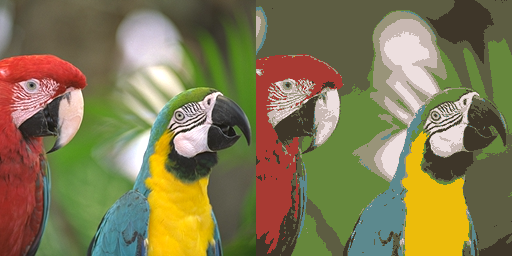The image on the right shows the result of K-means processing with 9 clusters. Since the number of clusters = the number of colors, the processed image is drawn in only 9 colors. The image looks like a slightly flat coloring book.

In the previous article, we used only pixel values as input for K-means, so if the colors were similar, pixels in a slightly distant area of the image would be divided into the same cluster.

So, I thought that if I clustered the pixel coordinate information in addition to the pixel value, I could get a path-like vector-like image, so I tried it.

## result

Here is the code I used.

``````import os
import argparse
import numpy as np
import cv2

if __name__ == "__main__":
#Specify the path as an argument
parser = argparse.ArgumentParser()
parser.add_argument('--img', '-i', help='The path of the image you want to process')
parser.add_argument('--output', '-o', help='Directory to output the result image')
args = parser.parse_args()

#Image as it is k-Since it cannot be applied to means, shape(Number of pixels, 3(BGR))Conversion to
Z1 = img.reshape((-1, 3))

"""
Get xy coordinates for each pixel
"""
#Vertical and horizontal width of the image
h, w = img.shape[:-1]
#Two-dimensional list of xy coordinates of images
Z2 = [[i, j] for i in range(h) for j in range(w)]

# RGB +Concatenate xy coordinates
Z = np.hstack((Z1, Z2))
# np.Convert to float32 type
Z = np.float32(Z)

# k-means termination condition
#Use default value
criteria = (cv2.TERM_CRITERIA_EPS + cv2.TERM_CRITERIA_MAX_ITER, 10, 1.0)
#Number of groups after split
for K in range(5, 21, 3):
# K = 10
# k-means processing
_, label, center = cv2.kmeans(
Z, K, None, criteria, 10, cv2.KMEANS_RANDOM_CENTERS)

# np.Convert to uint8 type
center = np.uint8(center)
res = center[label.flatten()]
res = res[:, :-2]
#Restore the original image size
res2 = res.reshape((img.shape))

output_path = os.path.join(args.output, 'Parrots_K{:02}.png'.format(K))
#Save image
cv2.imwrite(output_path, res2)
``````

Here are the main processes added to the previous code.

``````"""
Get xy coordinates for each pixel
"""
#Vertical and horizontal width of the image
h, w = img.shape[:-1]
#Two-dimensional list of xy coordinates of images
Z2 = [[i, j] for i in range(h) for j in range(w)]
``````

This part of the process gets the xy coordinates for each pixel. By combining the xy coordinates obtained here with the list of pixel values, a list that concatenates RGB + xy coordinates is generated. First, get the vertical width (h) and horizontal width (w) of img. The xy coordinate list is created with the line Z2 = ~ as [[0, 0], [0, 1], [0, 2] ...].

``````# RGB +Concatenate xy coordinates
Z = np.hstack((Z1, Z2))
``````

This line combines the list of pixel values and xy coordinates, and the data to be input to K-means is completed.

Below are the results. The original image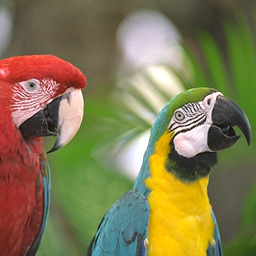K = 5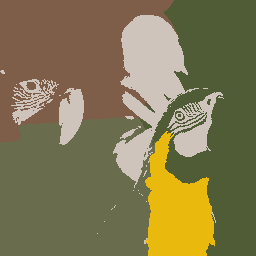K = 8K = 11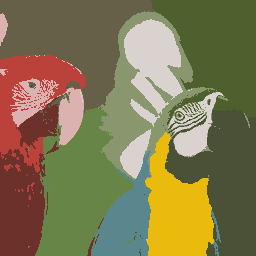K = 14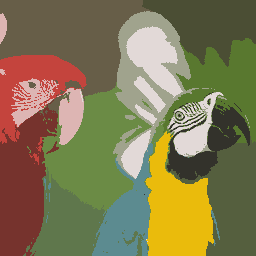K = 17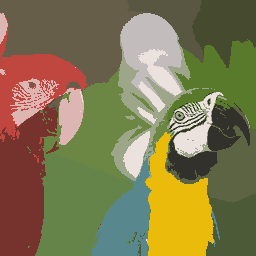K = 20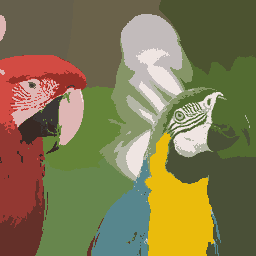Not only the pixel value but also the position is taken into consideration when dividing into clusters, so it looks like a path!

- Image </ b>